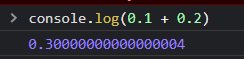# 0.1 + 2.8 ~= 1.1 + 1.8

You’re right, but floating-point errors are bound to happen. For example, running `0.1 + 0.2` in languages like Python or JavaScript, would return:1 Like

Unfortunately, these anomalies are ‘normal’ when it comes to floating-point and you will have to live with them.
You can use my ‘Round’ function described in this topic, to avoid this problem.

1 Like

Floating point errors were mentioned in a fairly recent Announcement about how Roblox was handling numbers.
I can’t remember which post it was, but maybe try searching Floating point error and search in the Announcements forum.

3 Likes

Also, when comparing float values with the equal operation you’ll have to use a small error of margin in your code. Example:

``````local A = 0.1 + 0.2
local B = 0.3
local err = 1e-6

print(math.abs(A - B) <= err)
``````
1 Like

Since nobody ever mentions it, this is why it happens. In our number system, base 10, what is the answer to 1/3?
The answer is 0.3333333333 repeating infinitely. We don’t have infinite space on the paper, so let’s round it off at 5 decimal places.
0.33333
Multiply by 3 again and you get 0.99999. it’s not 1.
This is a similar rounding error that exists in our every day base 10 number system.
What’s more is that 1/3*2 and 2/3 are different numbers.
(1/3 = 0.33333) * 2 = 0.66666
2/3 = 0.66667

In binary or base 2, this type of error caused by numbers repeating infinitely is a lot more common. Like tape measures in Inches, binary numbers work best when dividing by 2. Halves, quarters, eighths, sixteenths, thirty-seconds, sixty-fourths, etc. If your number can’t be represented with these inverse powers of two, it must get rounded.

5 Likes

I think that’s the simplest way to do it, thank you, but I hope Roblox staff can handle it for us. We can just focus on game development and not focus on the details

so mathematically on a base 10 number system youre right. Computers use binary. This is the product of that. That value is what value the computer is using. It shouldnt matter what a human thinks it should be, what matters is what the value ACTUALLY is for the machine.

1 Like
``````function Round(Number, Digits)

local Num = Number * 10 ^ Digits

Num = Num>=0 and math.floor(Num+0.5) or math.ceil(Num-0.5)

Num = Num / (10 ^ Digits)

return Num

end
``````

This method comes from @ rogeriodec_games, Should be built into Roblox as a friendly game engine

It is, math.round exists in Luau. You just need to handle the digit rounding:

``````local a = 0.13285358942
a = math.round(a * 1000) / 1000 --> 0.133
``````
3 Likes

I really don’t think you understand how the floating point error happens and why it happens as a byproduct. Do some research on it and you will understand why they can’t just “fix” it.

You’re right, I don’t really know the deep reasons, because it’s boring to study it，I just want to make games with intuitive functions

1 Like

Your method is more concise, I decided to use your method in my game, thank you1 Like

This is not just a double * double problem, it’s also a double * int problem.Sometimes, if we don’t pay attention to these details, it can have a big impact on our if-else logic

I would’ve done `string.format('%.3f', a)`

You can control the precision, plus you might be using stuff compiled from native C to perform the calculations.

I think the method you mentioned is really used a lot, and maybe the Roblox official want us to use this method to solve this problem, but I’m concerned about the performance, if I need real-time high-frequency calculation, for example, I’m shooting a monster, and I’m converting to string and then to double every time, Whether this performance cost is superfluous in the case of multiplayer online

1 Like

I have tested the string.format performance loss to be more than ten times the answer I adopted

``````local t = tick()
for i=1, 9999 do
if tonumber(string.format('%.3f',0.1 + 0.2))==0.3 then

end
end
print(tick() - t)

local t = tick()
for i=1, 9999 do
if math.round((0.1+0.2)*1000)/1000==0.3 then

end
end
print(tick() - t)
``````

Above is the code I tested, and below is the output

When I tested your solution in studio, there was no change. The function float didn’t work

Oh! I was thinking you were trying to print the string represenation of the number and not much else. In that case, I would recommend changing `1000` to `1e3`, or using `math.abs(0.1+0.2-0.3)<1e-3`

I ran this snippet:

``````local t = tick()
for i=1, 9999 do
if math.round((0.1+0.2)*1000)/1000==0.3 then

end
end
print(tick() - t)

local t = tick()
for i=1, 9999 do
if math.abs(0.1+0.2-0.3)<1/1000 then

end
end
print(tick() - t)
``````
``````0.00030851364135742190 VS 0.00023102760314941406
0.00030684471130371094 VS 0.00017261505126953125
``````

Performance difference is not negligible, and I recommend using the latter as you’re including epsilon only once.

This method has been established as the de-facto for professional programmers everywhere.

But I’ll go with the first function because I like functions that are straightforward and I’m afraid I’ll get a headache reading my own code after a long time.But thank you for your answer. I think someone else could use it.

1 Like